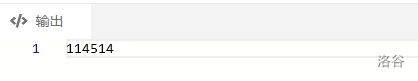# 浅谈STL容器：map

## 关于map

$map$ 的神奇之处在于，它的“下标”可以是任意数据类型，相当于加强的数组，本来数据的下标只可以是 $int$ 类型，但是依靠于 $map$ 的强大，我们可以用 $string$ 类型来做 $map$ の下标，不仅 $string$ ，甚至 $long long$$char$ 等等你能想到的任何数据类型，都可以作为他的下标,强大之处可见一斑

## 用法

#include<map>
#include<iostream>
#include<cstdio>
#include<cstring>
using namespace std;
map<string,int> x;
int main()
{
string s="Luogu";
x[s]=114514;
cout<<x[s];
}## 例题

LuoguAT899

#include<cstdio>
#include<iostream>
#include<map>
#include<cstring>//map和string的库名
using namespace std;
int n,sum,maxx=-114514;//maxx是用来求最大次数的
map<string,int> x;//map定义，前面的是下标类型，后面的是存的类型
string k,ans;//存名字
int main()
{
cin>>n;
for(int i=1; i<=n;i++)
{
string s;
cin>>s;
if(x[s]==0) sum++;//统计一共有几个名字存出现
x[s]++;//相应的map值++
k[i]=s;//存储下名字
}
for(int i=1; i<=sum;i++)//求出现次数最多的名字
{
if(x[k[i]]>maxx)//如果比他大，就更新最大值和最大值的名字
{
maxx=x[k[i]];
ans=k[i];
}
}
cout<<ans<<endl;//输出（记得换行）
return 0;
}


## 附带map的一些常用函数

  begin()          返回指向map头部的迭代器
clear(）         删除所有元素
count()          返回指定元素出现的次数
empty()          如果map为空则返回true
end()            返回指向map末尾的迭代器
equal_range()    返回特殊条目的迭代器对
erase()          删除一个元素
find()           查找一个元素
get_allocator()  返回map的配置器
insert()         插入元素
key_comp()       返回比较元素key的函数
lower_bound()    返回键值>=给定元素的第一个位置
max_size()       返回可以容纳的最大元素个数
rbegin()         返回一个指向map尾部的逆向迭代器
rend()           返回一个指向map头部的逆向迭代器
size()           返回map中元素的个数
swap()            交换两个map
upper_bound()     返回键值>给定元素的第一个位置
value_comp()      返回比较元素value的函数
posted @ 2021-06-04 18:09  Edolon  阅读(19)  评论(0编辑  收藏  举报
Live2D